# A Simple Model of Extraction

A little while ago, Jonathan Gagné suggested that I should take a look at the plot of extraction yield (EY) vs. brew ratio. His hypothesis was that we could fit a particular curve to the data in this plot which would give some insight into the extraction process.

## The model

When we think in very simple terms about the espresso extraction process, we might describe it like this:

We pass water through ground coffee in a portafilter. Each gram of water that passes through the coffee takes with it some of the remaining soluble compounds in the coffee.

When we have a process like this, where a portion of what remains is taken away in each moment, a physicist’s mind automatically goes to the exponential function. If we plot the amount of soluble coffee left in the portafilter as we pass water through it, we might expect something like this:

We can’t usually measure the amount of soluble coffee left in the portafilter, or the amount of water we’ve passed through it. But we can measure two things that are closely related: EY and brew ratio.

Extraction yield is really just a measure of how much soluble coffee winds up in the cup, scaled by how much dry coffee we started with. And brew ratio is just a measure of the total weight of the beverage, again scaled by the amount of dry coffee we started with.

We might expect the plot of EY vs. brew ratio to look like this:

This plot captures a lot of our intuition about the espresso extraction process. We expect the soluble coffee to come out quickly at first—the liquid coming out of the portafilter will be quite thick and dark—and then more slowly later—the liquid gets thinner and lighter in colour. If we let the shot run long enough, we would approach some “asymptotic” value of EY. In the language of math, our curve is: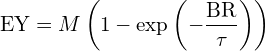Here,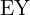is the extraction yield,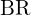is the brew ratio,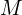is the asymptotic value of extraction yield, and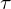is the time constant for the extraction—basically, how long it takes for 63% of the soluble coffee to enter the cup.

## Results

I set out to test Jonathan’s hypothesis right away. Every morning I make two shots of espresso, so what I did was to split each of these shots at a different beverage weight, then measure total dissolved solids (TDS) for the first part. Once I had taken a small sample from the first part, I combined the two and enjoyed some tasty espresso.

As usual, I’ve uploaded my results and analysis to Binder and GitHub, for anyone who wants to see the gory details.

Let’s see what the plot of EY vs. brew ratio looks like for a real espresso shot.

The best fit line uses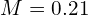and. To put this into plain language:

• If we let this shot run forever, it would never go higher than 21% extraction yield
• At a brew ratio of 0.63, we’ve extracted about 63% of the soluble coffee into the cup (the similarity of the two figures is a coincidence)

## Transforming the results

The breadth of this result didn’t really strike me until I thought about the relationship between TDS, EY, and brew ratio. To review, let’s define these three important values: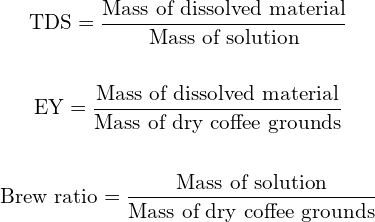This means that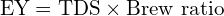If we know any two of these values, we can calculate the third using this equation. Because of this, we can transform our simple and intuitive model from the plot of EY vs. brew ratio above into the plot of TDS vs. EY:

Here, the best fit line has been transformed into a trajectory in the brewing control chart, defined by just two parameters:and. To emphasize the point, these are the same measurements and the same curve that we saw in the previous plot. All we’ve done is to transform them into a different set of coordinates.

The scale drawn along the trajectory indicates brew ratio, so we could use this plot to predict what brew ratio we would need to stop at in order to achieve a particular TDS and EY.

This plot also shows us what values of TDS and EY are achievable with this particular bean and equipment configuration. By repeating this experiment with different beans and equipment configurations, we could compare what is achievable with each.

## Error analysis

Early on, I realized that the data here has significant uncertainty both in TDS and in brew ratio. Because of this, I didn’t want to do a simple least squares fit. With a little help from Jonathan, who deals with similar issues all the time in his research, I wound up going down the rabbit hole of frequentism vs. Bayesianism.

Jake VanderPlas has an excellent series of articles discussing these two approaches to modeling experimental data. The distinction is still quite new to me, so I won’t try to summarize Jake’s articles here, but I think I may be able to do justice to a brief description of the difference between the two approaches.

Frequentism is the classical way of looking at data. Under this view, there are underlying “true” values for the model parameters. We then use our experimental data to get an estimate of those “true” values.

On the other hand, the Bayesian approach assumes that there is no underlying “true” value for the model parameters. Instead, we start with “prior” probabilities which describe what we know already about the model parameters, then we use new experimental data to update those probabilities through the process of Bayesian inference. These updated probabilities are called “posterior” probabilities.

The Bayesian approach is conceptually, and mathematically, a little more difficult, but I like the idea that with experiment, what we’re really doing is refining our beliefs about the system, not uncovering some underlying truth about objective reality.

In keeping with the Bayesian theme, I’ve used a tool called Markov chain Monte Carlo analysis to determine the uncertainty in our reported model parameters. This method uses discrete samples from our experimental data to estimate the posterior probabilities for our model parameters.

In terms of model parameter uncertainty, for the experiment shown here, we have the following 1-sigma uncertainties: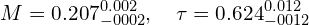Visually, the result looks like this:

The shaded region shows the 95% “credible interval” for the model parameters. Essentially, given our updated beliefs after incorporating the experimental data, 95% of the time the model parameters should fall within this range.

So why do so many points fall outside of that range?

The model I’ve used here accounts for measurement uncertainty—I’ve used an uncertainty of 0.05 g for weights and 0.2% Brix for refractometer measurements—but it doesn’t account for variation in the process itself. I think the most likely sources of process variation are:

• Variation in particle size distribution for the ground coffee
• Variation in pressure due to hysteresis in the over pressure valve in my Gaggia Classic Pro
• Variation in puck preparation

I’m working on adding process uncertainty to the model, but in the meantime I will say that these measurements have given me a lot of insight into the variations in my process. For example, it’s through these measurements that I’ve realized that—particularly with the 6.5 bar spring installed—variation in the response of the OPV can cause significant changes in shot timing.

## Conclusion

The reported parameter uncertainties should be taken with a grain of salt, since they do not take process variations into account. However, I would say this method has a lot of potential for comparing different beans, equipment configurations, and workflows.

Making these measurements has certainly helped me identify a few sources of variation in my process. I would encourage you to give it a try, and see what you learn about your own process.

I’ve provided an intuitive justification for the model presented here, but there is a mathematical justification as well. In an upcoming post, I will be outlining an analytic solution to a simplified version of the model presented by Moroney et al. in their 2019 paper, and we’ll see the same exponential form come up again.

1.Jeond dawoon says:

i have a question for the EY model.
i’m not familiar with physical aspect.
i can find a little close formula you used this blog, exponential decay
but it is not same as.
could you explain about EY model more detailed ?
how to derive model equation and how to compute parameter ‘M’ and ‘tau’
huge thank you.

1.Michael Cooper says:

The model presented in this post was not based on calculation from fundamental properties. Instead, we started with a high-level intuition about extraction, and chose a very simple model based on that.

In a previous post, I looked at the 1D model of Moroney et al. (2019):

https://quantitativecafe.com/2021/11/14/modeling-extraction/

As it turns out, we *can* derive the exponential model presented here as an analytic solution to Moroney’s 1D model in the special case where we model the packed bed as a single layer (and where the initial conditions meet certain requirements). I’ll be posting that derivation soon, but one thing I’d like to share now is that the parameters M and tau both seem to be affected by microscopic parameters in a complex way—I don’t think the fundamental properties of the bean divide nicely into one parameter or the other.

1.Jeond dawoon says:

thanks kindly and detailed answer !
i’m waiting for derivation of model.
and i have another question, the ‘tau’, do i understand that the higher tau is the slower flow rate ?
thank you !

1.Michael Cooper says:

Tau is the “time constant” for the exponential function in the extraction model. This will probably be related to flow rate, since a slower flow rate means more contact time between the liquid and solid phases, which means more extraction per gram of liquid. But it will also be related to grind size and properties of the bean–more surface area or a bean which extracts more easily will also result in more extraction per gram of liquid.